# Math in Focus Grade 6 Chapter 10 Review Test Answer Key

Practice the problems of Math in Focus Grade 6 Workbook Answer Key Chapter 10 Review Test to score better marks in the exam.

## Math in Focus Grade 6 Course 1 B Chapter 10 Review Test Answer Key

Concepts and Skills

Identify a base and a height of each triangle.

Question 1.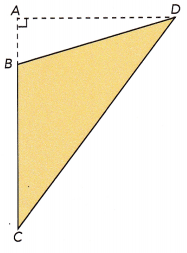Assume a side as the base of triangle and the perpendicular segment between the base and the opposite vertex will be the height of the triangle.
Let us assume the side BC as the base, then the segment AD which is drawn perpendicular between the base and the opposite vertex will be the height.
Thus, base of triangle = BC
Height of the triangle = AD.

Question 2.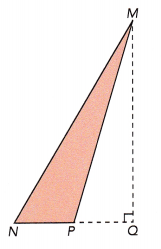Assume a side as the base of triangle and the perpendicular segment between the base and the opposite vertex will be the height of the triangle.
Let us assume the side NP as the base, then the segment MQ which is drawn perpendicular between the base and the opposite vertex will be the height.
Thus, base of triangle = NP
Height of the triangle = MQ

Find the area of each figure.

Question 3.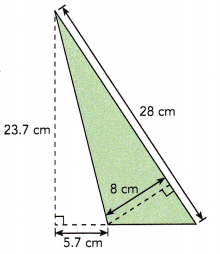If we assume the line segment measuring 28cm as the base, then the height will be the perpendicular line segment between the base and the opposite vertex which is 8cm.
Area of triangle = $$\frac{1}{2}$$×28×8
= $$\frac{1}{2}$$×224
= 224÷2
= 112 sq.cm
Thus, the area for the given polygon will be 112 sq.cm

Question 4.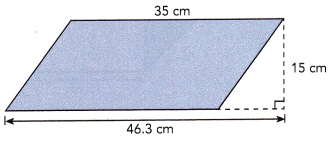The given polygon is parallelogram.
Let us assume the base of the parallelogram as 46.3 cm, then the height will be the perpendicular line segment between the base and the opposite vertex which is 15 cm.
Area of parallelogram = base×height
= 46.3×15
= 694.5 sq.cm
Thus, the area for the given polygon will be 694.5 sq.cm

Question 5.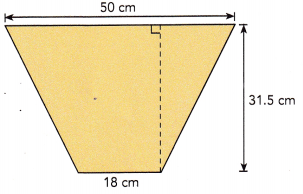The given polygon is similar to trapezium.
A trapezium has a pair of parallel sides, measuring 50cm and 18cm.
The height will be the perpendicular line segment between the base and the opposite vertex which is 31.5 cm.
Area of trapezoid = $$\frac{1}{2}$$×(sum of bases)×height
= $$\frac{1}{2}$$×(50+18)×31.5
= $$\frac{1}{2}$$×68×31.5
= 2142÷2
= 1071 sq.cm
Thus, the area for the given polygon will be 1071 sq.cm

Find the area of the shaded region.

Question 6.
The area of the regular octagon below is 560 square inches.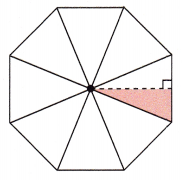A regular octagon will be made up of her 8 identical triangles.
Thus, the area of octagon will be equal to sum of the 8 identical triangles.
Given that the area of the regular octagon is 560 sq.in
Area of octagon = 8 × Area of triangle
560 = 8 × Area of triangle
560÷8 = (8 × Area of triangle)÷8
Area of triangle = 70sq.in
The shaded region is half of the triangle.
Area of shaded region = $$\frac{1}{2}$$×Area of triangle
= $$\frac{1}{2}$$×70
= 35 sq.in
Thus, the area of shaded region is 35 sq.in

Problem Solving

Find the area of the shaded region.

Question 7.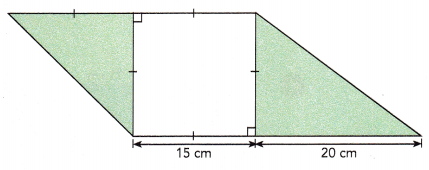The given figure has two triangles and a square.
Length of square is 15 cm.
Area of square will be length×length
= 15×15
= 225 sq.cm
The area of shaded region can be calculated by subtracting the area of square from the area of parallelogram.
So, calculate the area of parallelogram.
Area of parallelogram = base×height
The base of parallelogram is 15+20=35 cm and
the height will be 15 cm.
= 35×15
= 525 sq.cm
Area of shaded region = Area of parallelogram – Area of square
= 525 – 225
= 300 sq.cm

Solve.

Question 8.
Figure ABCD is a parallelogram. BC is 16 centimeters, CD is 12 centimeters, and AH is 10 centimeters.
a) Find the area of parallelogram ABCD.
Given that figure ABCD is a parallelogram.
BC is 16 centimeters and AH is 10 centimeters.
Area of parallelogram ABCD = base × height
= BC × AH
= 16×10
= 160 sq.cm

b) Find the length of $$\overline{\mathrm{AK}}$$. Round your answer to the nearest tenth of a centimeter.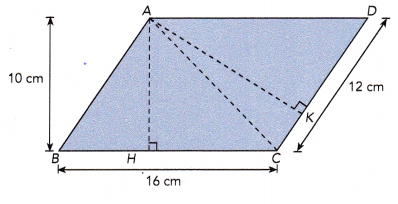ABCD is a parallelogram with two triangles ABC and ACD.
Area of parallelogram = Area of triangle ABC + Area of triangle ACD
The area of parallelogram is 160 sq.cm
Area of triangle ABC= $$\frac{1}{2}$$×base×height
= $$\frac{1}{2}$$×BC×AH
= $$\frac{1}{2}$$×16×10
= 160÷2
= 80 sq.cm
Area of parallelogram = 80 + Area of triangle ACD
160 = 80 + Area of triangle ACD
160-80 = 80 + Area of triangle ACD – 80
80 = Area of triangle ACD
Area of triangle ACD = $$\frac{1}{2}$$×base×height
80 = $$\frac{1}{2}$$×12×AK
80 = 6×AK
80÷6 = (6×AK)÷6
13.3 = AK
13.3 when rounded of to nearest tenth will be 13
The length of AK = 13cm

Question 9.
Figure ABCD is a trapezoid. The length of $$\overline{\mathrm{BC}}$$ is 36 centimeters. The areas of triangles ABC and ACD are in the ratio 1.5:1. Find the length of $$\overline{\mathrm{AD}}$$.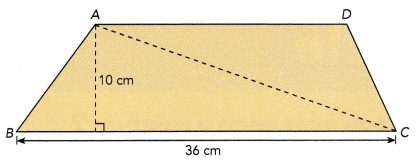Given that figure ABCD is a trapezoid.
The length of $$\overline{\mathrm{BC}}$$ is 36 centimeters.
Area of triangle ABC = $$\frac{1}{2}$$×base×height
= $$\frac{1}{2}$$×36×10
= 360÷2
= 180 sq.cm
The areas of triangles ABC and ACD are in the ratio 1.5:1
$$\frac{ABC}{ACD}$$ = $$\frac{1.5}{1}$$
Area of triangle ABC = 1.5×Area of triangle ACD
180 = 1.5×Area of triangle ACD
180÷1.5 = (1.5×Area of triangle ACD)÷1.5
120 = Area of triangle ACD
Area of triangle ACD = $$\frac{1}{2}$$×base×height
120 = $$\frac{1}{2}$$×AD×10
Thus, the length of $$\overline{\mathrm{AD}}$$ is 24 cm.

Question 10.
Parallelogram PRTVis made up of triangle PQV, triangle QUV, and trapezoid QRTU. The area of parallelogram PRTV is 96 square feet. The lengths of $$\overline{\mathrm{TU}}$$ and $$\overline{\mathrm{UV}}$$ are equal. Find the area of triangle QUV.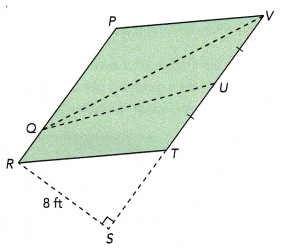Parallelogram PRTV is made up of triangle PQV, triangle QUV, and trapezoid QRTU.
The area of parallelogram PRTV is 96 sq.ft. The lengths of TU is equal to UV.
Area of parallelogram PRTV = 96
Area of parallelogram PRTV = base×height
96 = base×8
96÷8 = (base×8)÷8
12 = base
TV is the base of the parallelogram PRTV which is equal to 12 ft.
TV = VU+UT
12 = VU+VU
12 = 2×VU
12÷2 = (2×VU)÷2
6 = VU
Length of VU is 6 ft.
Area of triangle QUV = $$\frac{1}{2}$$×base×height
= $$\frac{1}{2}$$×UV×8
= $$\frac{1}{2}$$×6×8
= 48÷2
= 24 ft

Question 11.
Charles drew a regular hexagon and divided it into two identical trapezoids. The side length of the hexagon is 16 centimeters, and the length of the diagonal shown is 32 centimeters. Charles measured the height of one of the trapezoids and found that the height was 13.9 centimeters. Find the area of the hexagon.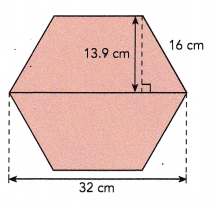A regular hexagon and divided it into two identical trapezoids.
The side length of the hexagon is 16 cm and the height of one of the trapezoids is 13.9 cm. The length of the diagonal shown is 32 cm.
The side length makes with the height of the trapezium.
Pythagoras theorem is applied to get the base of the triangle.
base² = 16²-(13.9)²
base² = 256-193.21
base² = 62.79
base will be the square root of 62.79
Base length will be 7.9 cm
The length of opposite diagonal can be calculated by subtracting the sum of base length of two triangle from the length of diagonal.
Length of the parallel side = 32-(7.9+7.9)
= 16.2 cm
Area of trapezoid = $$\frac{1}{2}$$×(sum of bases)×height
= $$\frac{1}{2}$$×(32+16.2)×13.9
= $$\frac{1}{2}$$×48.2×13.9
= $$\frac{1}{2}$$×669.9
= 334.9 sq.cm
Area of hexagon = Area of trapezoid + Area of trapezoid
= 334.9+334.9
= 669.8 sq.ft
Thus, the area of hexagon is 669.8 sq.ft